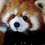# A new derivative

We can define a logarithmic derivative of a function $f(x)$ as $f^\ddagger (x) = \frac{d}{dx} ln \; f(x) = \frac{f'(x)}{f(x)}$

It's easy to see that it takes on a few nicer properties than derivatives typically do when it comes to quotients and composition

$(fg)^\ddagger = f^\ddagger + g^\ddagger$ $(f/g)^\ddagger = f^\ddagger - g^\ddagger$ $(f(g))^\ddagger = f^\ddagger (g) g'$

We can define common derivatives in terms of the logarithmic derivative:

$(x^n)^\ddagger = n \frac{1}{x}$ $(e^x)^\ddagger = 1$ $(cos(x))^\ddagger = -tan(x)$ $(tan(x))^\ddagger = tan(x) + cot(x)$

Can you find a function $f(x)$ such that $f^\ddagger (x) = f(x)$?Note by Levi Walker
2 years, 8 months ago

This discussion board is a place to discuss our Daily Challenges and the math and science related to those challenges. Explanations are more than just a solution — they should explain the steps and thinking strategies that you used to obtain the solution. Comments should further the discussion of math and science.

When posting on Brilliant:

• Use the emojis to react to an explanation, whether you're congratulating a job well done , or just really confused .
• Ask specific questions about the challenge or the steps in somebody's explanation. Well-posed questions can add a lot to the discussion, but posting "I don't understand!" doesn't help anyone.
• Try to contribute something new to the discussion, whether it is an extension, generalization or other idea related to the challenge.

MarkdownAppears as
*italics* or _italics_ italics
**bold** or __bold__ bold
- bulleted- list
• bulleted
• list
1. numbered2. list
1. numbered
2. list
Note: you must add a full line of space before and after lists for them to show up correctly
paragraph 1paragraph 2

paragraph 1

paragraph 2

[example link](https://brilliant.org)example link
> This is a quote
This is a quote
    # I indented these lines
# 4 spaces, and now they show
# up as a code block.

print "hello world"
# I indented these lines
# 4 spaces, and now they show
# up as a code block.

print "hello world"
MathAppears as
Remember to wrap math in $$ ... $$ or $ ... $ to ensure proper formatting.
2 \times 3 $2 \times 3$
2^{34} $2^{34}$
a_{i-1} $a_{i-1}$
\frac{2}{3} $\frac{2}{3}$
\sqrt{2} $\sqrt{2}$
\sum_{i=1}^3 $\sum_{i=1}^3$
\sin \theta $\sin \theta$
\boxed{123} $\boxed{123}$

Sort by:

Yes.........there are infinitely many functions.......You need to specify the boundary conditions for a unique solution........Otherwise, it is a simple differential equation......

- 2 years, 7 months ago

It's just an exercise in finding a solution, not the solution :)

- 2 years, 7 months ago

Ohh.........yup.....didn't see that...:P

- 2 years, 7 months ago

Would be nice if you changed the notation for the logarithmic derivative... maybe $f_{\text{L}}(x)$ or $Lf(x)$. As Aaghaz has said somewhere else, a solution would come from a family of solutions to the differential equation $y' = y^2$. Have a look here for more information on this stuff.

- 2 years, 7 months ago

I've seen $\ddagger$ used, so I just adopted that notation. I also find it more aesthetically pleasing tbh, and we all know I'm a sucker for aesthetics.

- 2 years, 7 months ago